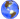# historic phases of the Heim theory

by von Ludwiger, Posdzech

 since 1949 m = m(r) Extension of the Newton's gravitation lawConsideration of field mass of a gravitational field 1952 non hermitian uniform field theory in R4 4-dimensional metric eigenvalue equations 1953 hermitian uniform field theory in R6 6-dimensional metric eigenvalue equations 1959 Theory of metron-geometry in R6 6-dimensional selector eigenvalue equations 1961 hermeneutic theory of possible structure condensations in space (Hermetrie) World selector equation (world formula) - it examines whether a theroretical structure may be item of physical world or not Vol 1  "elementary structures of matter" 1963  1964 beginning of the world, Trinity of Spheres,  nine interacting geometries polymetric 6-dimensional selector eigenvalue equations 1966 algebra of metric exchange processes in space Which interior dynamics can space possess? 1969 fundamental symmetries (baryon number, isospin) and 4 symmetries of higher order 1970 mass formula of elementary particles derived from internal geometrical characteristics of  space itself Vol 2  "elementary structures of matter"  (1984) 1962 effect of absolute Cartesian coordinates x5 and x6 Syntrometric method of a formal multi valued logic quantitative term structures describe processes in x5 and x6 1971 transition from geometry of metrons into a geometry of Syntrixes up to a totality of metroplexes (6) internal regularities of complex structures 1979 indirect conclusion about dynamics in added totalities of Metroplexes T(n):  Physis n = 3... 7  Bios n = 7 ... 16  Psyche n = 15 ... 24  Pneuma n = 25 ... How can these regularities look like?  How do they work within the area of the bodies? Which laws work in organisms?  Which laws work in psyche?  Which laws work in spirit? Band 3 "structures of physical world and its not-material background" (1997) Construction of a hyperspace R12 with not-material coordinates x7 ... x12 first approximation to structures of the not-material world 1994 controlling of the material world from not-material world by projection chains from G4 into the microbe realm of R6 Derivation of quantum mechanic laws (eigenvalue equations) as result of this controlling process investigation of the cosmological equation: effects of original natural set of numbers Derivation of todays physical coupling constants from the origin of the world cosmogony (development of the universe) age, distribution and movement of matter since 1997 1997 investigations about the temporal duration of existence of elementary structures

 You are visiting our website:  Wrld ITC.org       To reach our homepage click here please.